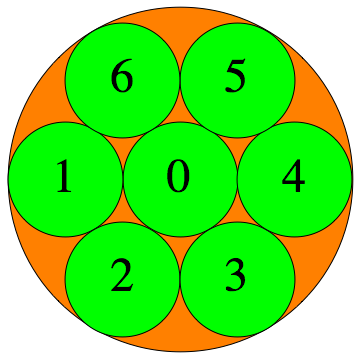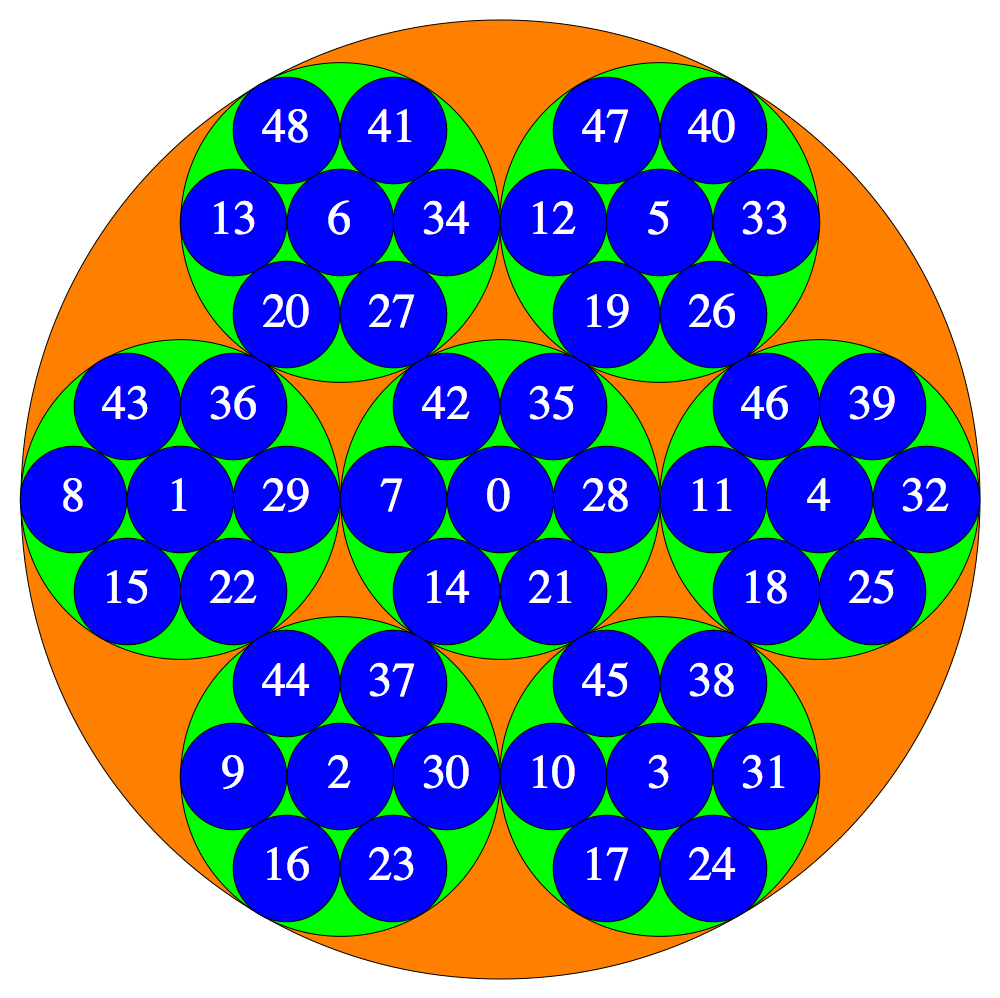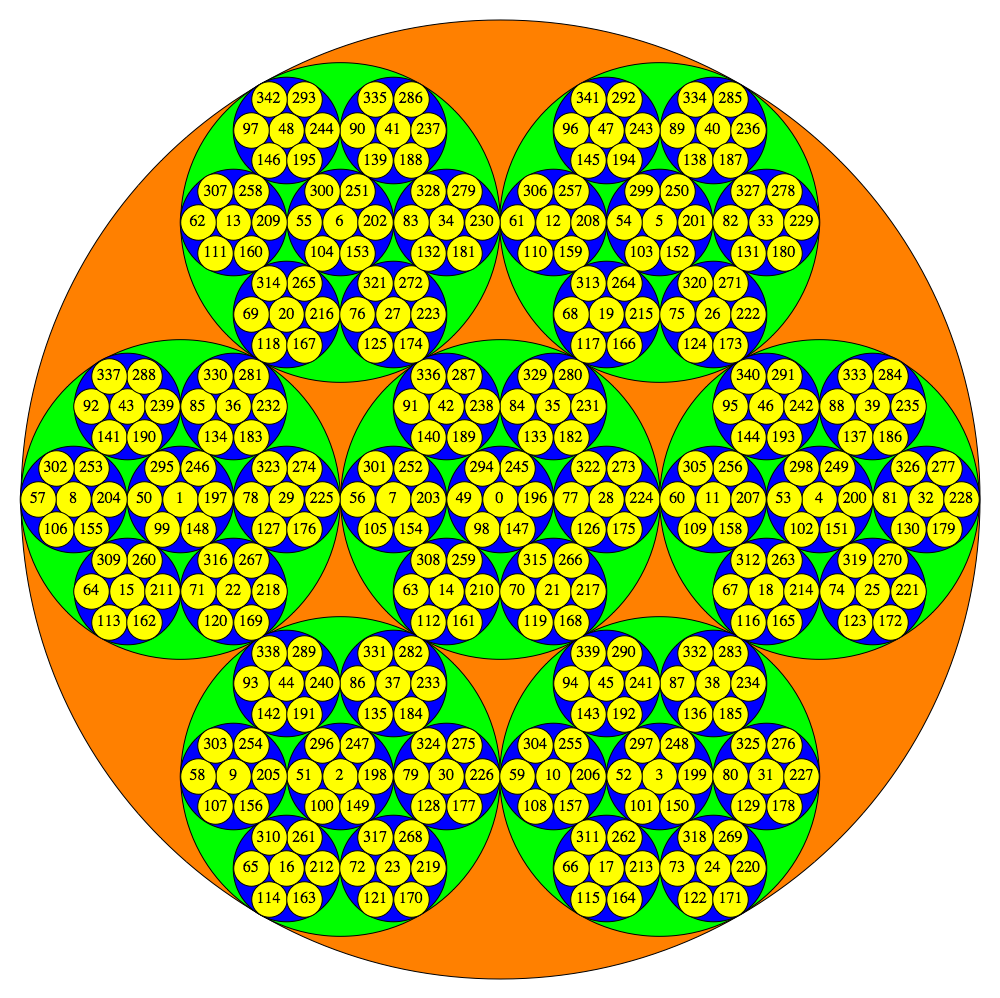# The p-adic integers and their topology

Illustrations by Heiko Knospe

The $p$-adic integers $\mathbb{Z}_p = \varprojlim \mathbb{Z}/p^n\mathbb{Z}$ are a compact, totally disconnected Hausdorff space, i.e., a Stone space. The figures show the clopen (closed and open) subsets $a+p^n \mathbb{Z}_p$ for $p=3, 5, 7$ and $n=1,2,3,\dots$

### $p=3$ :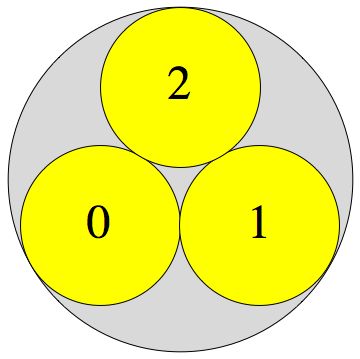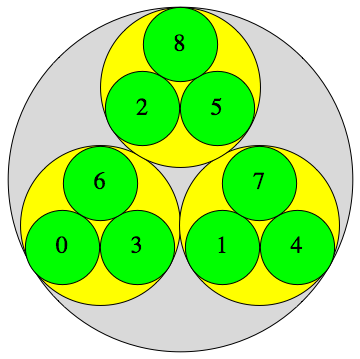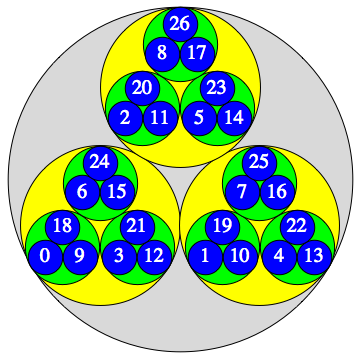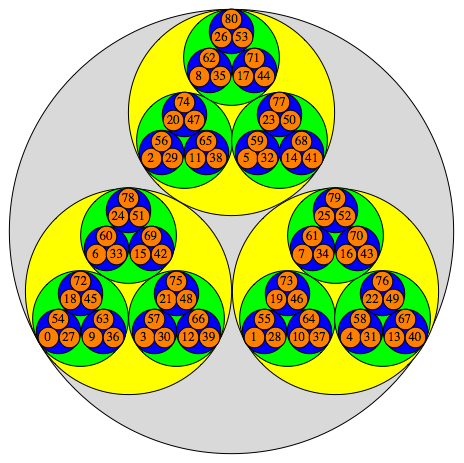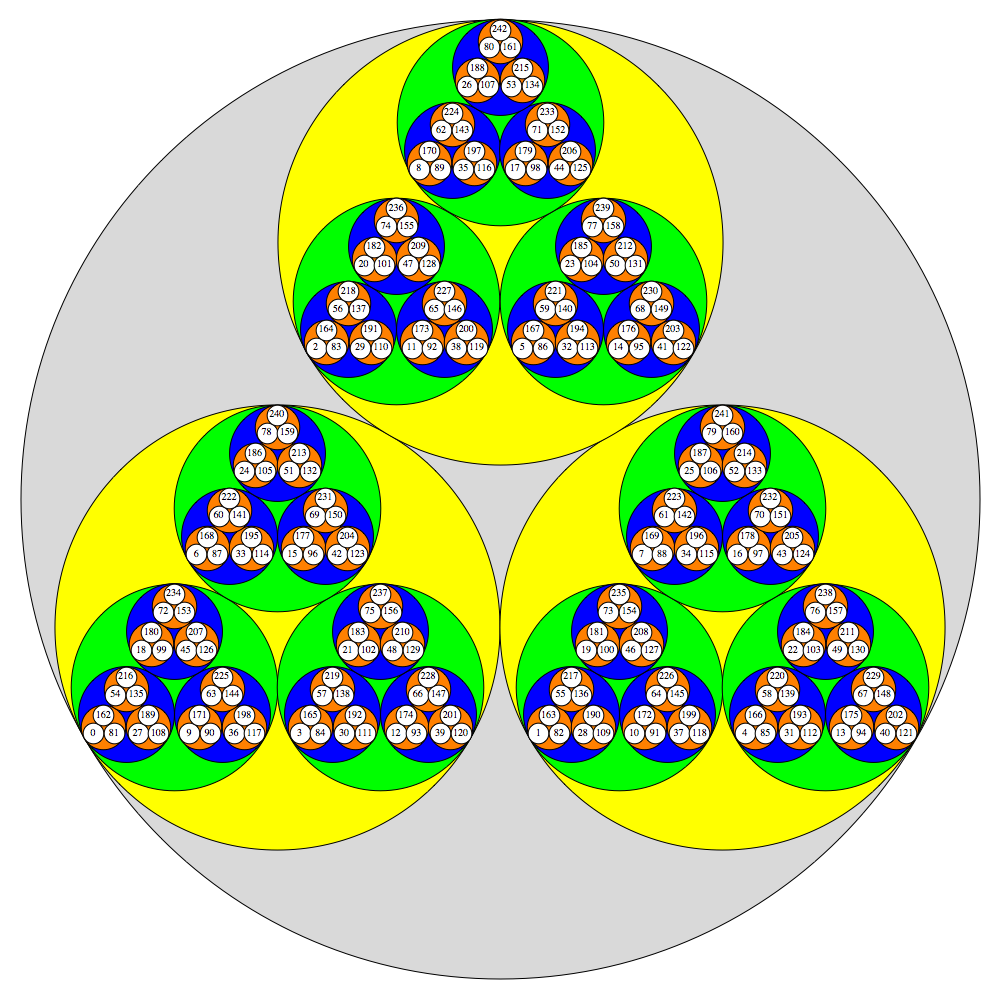### $p=5$ :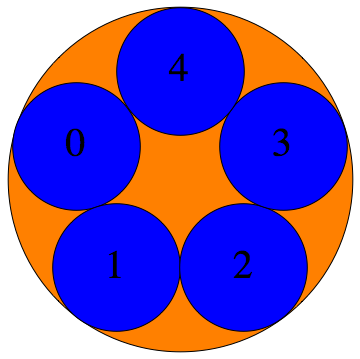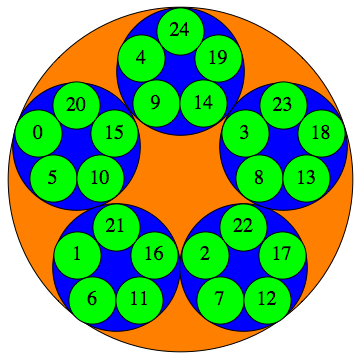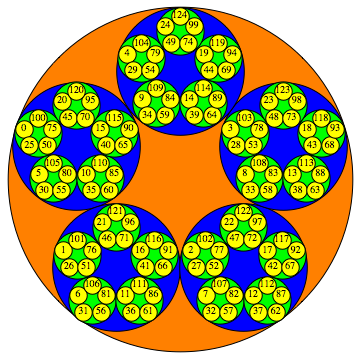### $p=7$ :